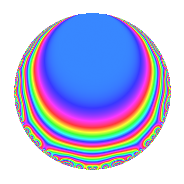# Properties

 Label 500.1.jLevel 500 Weight 1 Character orbit j Rep. character $$\chi_{500}(51,\cdot)$$ Character field $$\Q(\zeta_{10})$$ Dimension 4 Newforms 1 Sturm bound 75 Trace bound 0

# Related objects

## Defining parameters

 Level: $$N$$ = $$500 = 2^{2} \cdot 5^{3}$$ Weight: $$k$$ = $$1$$ Character orbit: $$[\chi]$$ = 500.j (of order $$10$$ and degree $$4$$) Character conductor: $$\operatorname{cond}(\chi)$$ = $$100$$ Character field: $$\Q(\zeta_{10})$$ Newforms: $$1$$ Sturm bound: $$75$$ Trace bound: $$0$$

## Dimensions

The following table gives the dimensions of various subspaces of $$M_{1}(500, [\chi])$$.

Total New Old
Modular forms 52 28 24
Cusp forms 12 4 8
Eisenstein series 40 24 16

The following table gives the dimensions of subspaces with specified projective image type.

$$D_n$$ $$A_4$$ $$S_4$$ $$A_5$$
Dimension 4 0 0 0

## Trace form

 $$4q + q^{2} - q^{4} + q^{8} - q^{9} + O(q^{10})$$ $$4q + q^{2} - q^{4} + q^{8} - q^{9} + 2q^{13} - q^{16} + 2q^{17} - 4q^{18} - 2q^{26} - 2q^{29} - 4q^{32} + 3q^{34} - q^{36} - 3q^{37} - 2q^{41} + 4q^{49} + 2q^{52} - 3q^{53} + 2q^{58} - 2q^{61} - q^{64} + 2q^{68} + q^{72} + 2q^{73} - 2q^{74} - q^{81} + 2q^{82} + 3q^{89} + 2q^{97} + q^{98} + O(q^{100})$$

## Decomposition of $$S_{1}^{\mathrm{new}}(500, [\chi])$$ into irreducible Hecke orbits

Label Dim. $$A$$ Field Image CM RM Traces $q$-expansion
$$a_2$$ $$a_3$$ $$a_5$$ $$a_7$$
500.1.j.a $$4$$ $$0.250$$ $$\Q(\zeta_{10})$$ $$D_{5}$$ $$\Q(\sqrt{-1})$$ None $$1$$ $$0$$ $$0$$ $$0$$ $$q-\zeta_{10}^{4}q^{2}-\zeta_{10}^{3}q^{4}-\zeta_{10}^{2}q^{8}+\cdots$$

## Decomposition of $$S_{1}^{\mathrm{old}}(500, [\chi])$$ into lower level spaces

$$S_{1}^{\mathrm{old}}(500, [\chi]) \cong$$ $$S_{1}^{\mathrm{new}}(100, [\chi])$$$$^{\oplus 2}$$